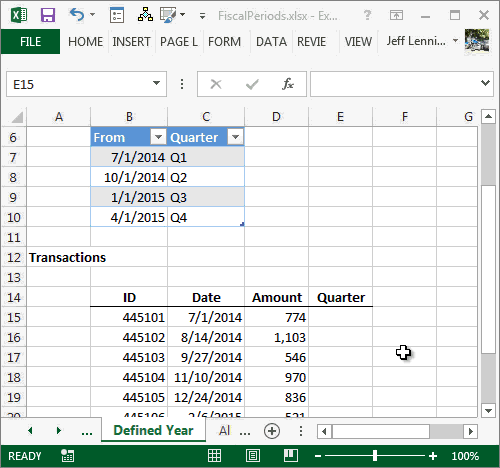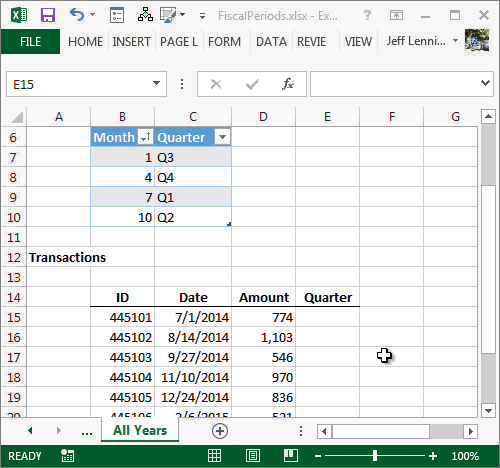## Create Fiscal Year Periods with VLOOKUP

I’m a huge fan of the VLOOKUP function, and am surprised by its day-to-day utility for accountants. In this post, we use the VLOOKUP function to convert or translate calendar year transaction dates into fiscal year periods, such as a fiscal quarter. To accomplish this, we’ll first need to investigate in detail the function’s fourth argument, the range lookup argument. Let’s begin.

## Range Lookup Argument

The syntax for the VLOOKUP function follows:

`=VLOOKUP(lookup_value, table_array, col_index_num, [range_lookup])`

Where:

• lookup_value – the value to find
• table_array – the range in which we are looking
• col_index_num – the column that has the value to return
• [range_lookup] – true or false, are we doing a range lookup?

The fourth argument, the optional range_lookup value, is the argument that enables us to easily translate dates into fiscal quarter groups, or, perform any other range lookup. So, what is a range lookup? It means we are trying to find a value between two endpoints. The word range here doesn’t refer to a worksheet range, such as A1:B10. It refers to a range between two values, such as a number between 1 and 100, 101 and 200, or in the case of dates, between 1/1/14 and 3/31/14. We are trying to find our lookup value within a range of values. Since this argument is optional, Excel users are not required to actually express a value…that is…we can leave it out of the function. The default value, if omitted, is TRUE, as in, true, we are doing a range lookup. The value of FALSE means we are not doing a range lookup, we want an exact matching value. In our case, we want to do a range lookup and so we’ll use TRUE for our formula.

Let’s give it a try.

## Simple Version – Specific Year

Before we write our formula, let’s be clear about our task. We have a list of transactions. Each transaction record includes the transaction date. We need to summarize the transactions, but our company is on a 6/30 fiscal year-end. Since Excel only supports calendar year date groups, we’ll need to use the VLOOKUP function to translate the transaction dates into fiscal period groups. To prepare our worksheet, we set up a little lookup table that Excel can use to make the translation. While we are getting warmed up, we can visualize the little lookup table as follows:We have a from date, a to date, and a quarter label. We’ll look up the transaction date in the table to determine the quarter label.

Assuming our first transaction date was stored in cell C15 and that the little lookup table is named Table1, then the following formula would work:

`=VLOOKUP(C15, Table1, 3, TRUE)`

Where:

• C15 is the lookup value, the transaction date
• Table1 is the lookup range, the quarter table
• 3 is the column that has the value to return, the quarter label, which is the third column in the table
• TRUE we are doing a range lookup

The results are shown below:The formula essentially finds the date in the lookup range and returns the quarter label.

But, we can do even better. When the VLOOKUP is finding the transaction date, it is really only looking in the first column, the “from” column, known as the lookup column. That means that it ignores the second column, the “to” column. Even though humans really love to see both date endpoints, Excel doesn’t need them. So, we can simplify our lookup table by excluding the “to” column, as shown below:This works because the VLOOKUP function only looks for its matching value in the first column, the lookup column, and ignores other columns. After a match is found, then the function slides to the right to return a related result, in our case, the quarter label.

Quick question for you: does the quarter lookup table need to be sorted? Yes, if the fourth argument of the VLOOKUP is TRUE, then the lookup range must be sorted in ascending order by the lookup column for it to return a reliable result.

## Advanced Version – All Years

We can do even better than the formula above. The lookup table above works for a specific fiscal year, but requires us to add new rows each subsequent year. Since our goal is to eliminate manual steps from recurring processes, we’ll need to figure out a way to create a lookup table that works for all years.

Fortunately, Excel provides the MONTH function which pulls the month number out of a date. By nesting the MONTH function in our VLOOKUP function, we can simplify our lookup table to include only months, as shown below.We can use the MONTH function to pull the month out of the date, as shown below:Remember, the lookup table needs to be sorted in ascending order for the function to return a reliable result. Since this table includes months only, it can be used for transactions that span many fiscal years.

And that my friend is my preferred method for translating transaction dates into fiscal periods. If you have other methods, please share by posting a comment below…thanks!

## Additional Resources

• Sample File: FiscalPeriods
• Tables: this post relies on the table feature…if you haven’t played with it yet check out the table related blog posts

Posted in ,### Jeff Lenning

I love sharing the things I've learned about Excel, and I built Excel University to help me do that. My motto is: Learn Excel. Work Faster.

### Stay Connected

If you'd like to be notified when I write a new Excel article, enter your name and email and click SUBSCRIBE. You can unsubscribe anytime, and I will never sell your email address.

### Want to learn Excel?

Our Campus Pass includes access to our entire Undergrad and Masters catalog. Gamification ensures it is the most fun you can have learning Excel :)

### 11 Comments

1.Zaigham on November 3, 2014 at 10:10 am

Nice explanation and demonstration. Keep it up.

•jefflenning on November 3, 2014 at 10:28 am

Thanks!

2.Mike on February 9, 2015 at 4:41 pm

Jeff,

How do you attach the Year to the output. I can’t just have “Q1”. I need “Q1 ’15”. Any help here?

•jefflenning on February 9, 2015 at 4:46 pm

Mike,
Sure…all you would need to do is update the values in the Fiscal Period Table to include the desired labels, for example, change the table values from Q1, Q2, Q3, and Q4, to include the year, such as Q1 2015, and so on.
Hope it helps!
Thanks
Jeff

3.Alison Perry on May 21, 2015 at 4:50 pm

Jeff
I have one spreadsheet with a date of 26/06/2015 but when I do a Vlookup in a different spreadsheet the date comes back as 30/06/2019.
Thanks
Alison

•Kurt LeBlanc on August 17, 2016 at 8:59 am

Hey Alison,

It sounds like there’s a formatting issue somewhere. I’m not sure, but I think one of the cells is formatted to display results in an unintended way…

Check that and let me know how it goes:)
Kurt LeBlanc

4.Alex Schwarzer on November 13, 2015 at 8:23 am

Very clear explination! Has helped me simpllfy the fiscal year juggling act!

You mention the advanced option as not having to add new rows. I don’t quite understand how this is avoided. As your ledger moves forward (ie, “grows” in time), won’t new rows have to be added as time advances? That would be in either scenario.

Maybe I’m missing it! Great advice and help – but would love some clarification how to avoid extra manual steps!

Thanks!

•jefflenning on November 13, 2015 at 8:29 am

Alex,
Glad to help! You won’t need to update the table rows in the advanced version because the only element considered is the month, and not the year or day. That means that your lookup table need only contain four rows. For a working sample of this technique, download the sample Excel file and check out the All Years worksheet.
Hope it helps!
Thanks
Jeff

5.alice on January 27, 2016 at 6:16 pm

Great thanks problem solved !

6.Ron MVP on March 7, 2018 at 2:41 pm

I tried to create a lookup using the “simple” method you described. I defined an “employee” table, and “contribution” lookup table.

I could make the lookup work when I used a column number ie
=VLOOKUP(C3,contributiontb,3,TRUE)

But when I used point and click to identify the lookup column it generated this forumula
=VLOOKUP(C3,contributiontb,contributiontb[@contrib],TRUE)

Which looks OK to me. But it gives me a few correct answers, and a bunch of #value! and #ref! errors

I have the sample file in OneDrive:
https://1drv.ms/x/s!Am8lVyUzjKfphnur0_03fkHV-GgM

Any suggestions would be appreciated.

•Jeff Lenning on March 8, 2018 at 11:57 am

Hi Ron,
So, the third argument of VLOOKUP is expecting an integer value. So, using the structured table reference contributionstb[@contrib] instead confuses VLOOKUP and yields the unexpected results. But, I think what you wanted to do is update the lookup value argument instead, which is the first argument. I think the formula you are after is more like this:
=VLOOKUP(contributiontb[@contrib],contributiontb,3,TRUE)
Hope it helps!
Thanks
Jeff

### Learn by Email

###### Subscribe to Blog (free)
!
!
Something went wrong. Please check your entries and try again.